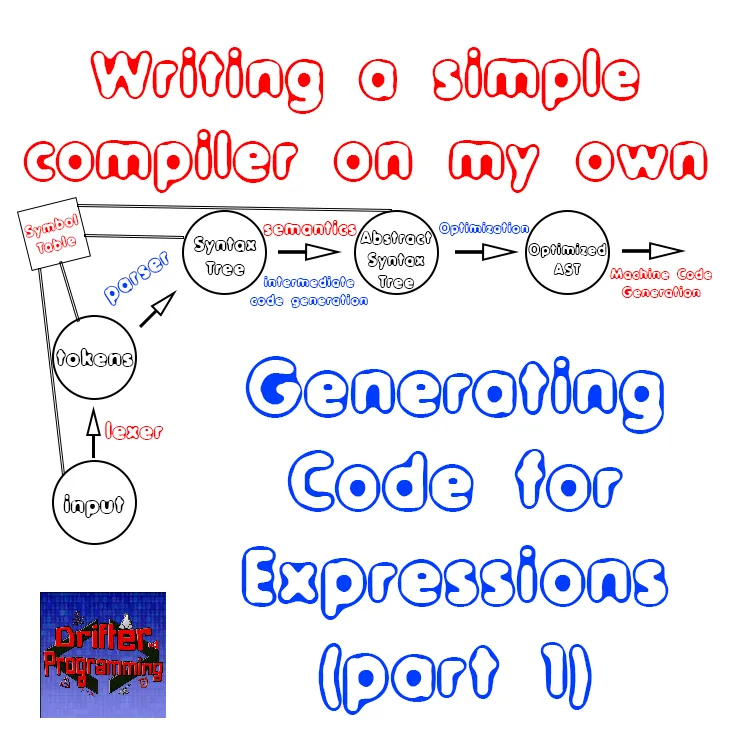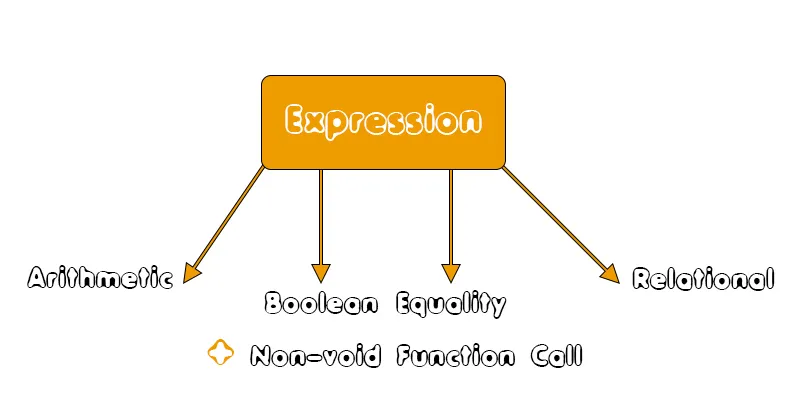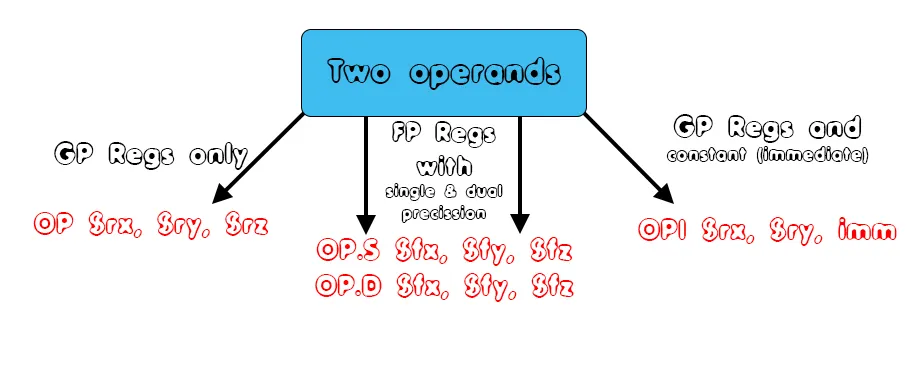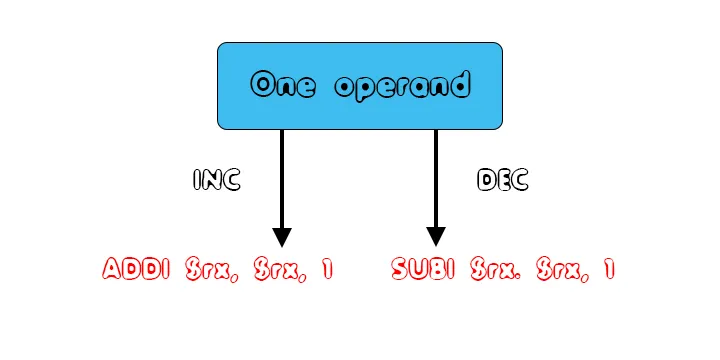# Writing a simple Compiler on my own - Generating Code for Expressions (part 1)[Custom Thumbnail]

All the Code of the series can be found at the Github repository:

https://github.com/drifter1/compiler

# Introduction

Hello it's a me again @drifter1! Today we continue with my Compiler Series, a series where we implement a complete compiler for a simple C-like language by using the C-tools Flex and Bison. In this article we will start with the Generation of Assembly Code for Expressions, covering most of the expression types and what has to be implemented in the next parts, through diagrams and an extensive theoretical explanation...

## Requirements:

Actually you need to read and understand all the topics that I covered in the series as a whole, as these articles will give you access to knowledge about:
• What Compiler Design is (mainly the steps)
• For which exact Language the Compiler is build for (Tokens and Grammar)
• How to use Flex and Bison
• How to implement a lexer and parser for the language using those tools
• What the Symbol Table is and how we implement it
• How we combine Flex and Bison together
• How we can pass information from the Lexer to the Parser
• How we define operator priorities, precedencies and associativity
• What Semantic Analysis is (Attributes, SDT etc.)
• How we do the so called "Scope Resolution"
• How we declare types and check the type inter-compatibility for different cases that include function parameters and assignments
• How we check function calls later on using a "Revisit queue", as function declarations mostly happen after functions get used (in our Language)
• Intermediate Code Representations, including AST's
• How we implement an AST (structure and management)
• Action Rules for AST nodes and more
• The target system's architecture and its assembly language (MIPS)
• Register Allocation & Assignment and how we implement it
• How we generate Assembly code for various cases

## Difficulty:

Talking about the series in general this series can be rated:
Today's topic(s) can be rated:
• Medium

# Actual Tutorial Content

## Expression Types

Taking a look at the "expression"-rule again, we can recall that our language allows the following expressions:
• Arithmetic
• expr ADDOP expr [+, - operators]
• expr MULOP expr [* operator]
• expr DIVOP expr [/ operator]
• ID INCR or INCR ID [++, -- operators]
• Boolean
• expr OROP expr [|| operator]
• expr ANDOP expr [&& operator]
• NOTOP expr [! operator]
• Equality - expr EQUOP expr [==, != operators)]
• Relational - expr RELOP expr [>, <, >=, <= operators]
• Parenthesis - (expr)
• Variable reference - var_ref
• constant (with or without sign) - (ADDOP) constant
• Function call (non-void) - function_call

Depending on the point where they occur, Boolean operations are not always taken into account as actual operations between registers, but their operands might be used in branch or set instructions instead. That's similar to how we use the Relational and Equality operations. But, we will still create a function that generates such expressions in Assembly Code.

Constants are quite easy to take care of and so after the various operators we will only have to talk about non-void function calls as parts of expressions (that will be generated way way later in the series) and how we will take care of variable references. In this final point we will basically get into the need of type conversion and movement between FP and GP registers.

So, the expressions that are important can be summarized as:## Arithmetic Operations

The most occurring operators in code are those that are used for calculations. Of course we are talking about the arithmetic operators. As we saw in one of the Register Allocation articles, arithmetic operations are of two formats
1. Two operands
2. One operand

The operands of an arithmetic expression can be stored inside of GP or FP registers, or be constants that are taken as immediate values, and so we can summarize two operands asReplacing OP with ADD, SUB, MUL and DIV we basically end up with the 4 basic arithmetic operations.

So, whenever both operands are integers or characters we use a simple OP instruction between GP registers. On the other hand, when one operand is stored inside of a GP registers and the other is a constant-immediate we use a OPI instruction. When either of the operands is a floating point value (constant or variable, doesn't matter) we have to use floating point instructions. To keep things simple we will only use OP.D instructions, supposing that floats are also doubles.

In our language we also have arithmetic operations that are used for incrementing and decrementing values. Those operations can be easily translated into ADDI or SUBI instructions. Thus, the one operand cases of arithmetic operations are:## Boolean Operations

Similar to Arithmetic operations, Boolean operations also have two or one operands. The AND and OR operations require two operands, whilst the NOT operation gets applied on one operand. The cases of instructions can be summarized by the same diagram as in arithmetic operations as the operands can again be GP (storing integers-characters), FP (storing floats-doubles) registers and constants-immediate values.

But, we are still not finished. Boolean operators work like that when they occur inside of Boolean operations that are used for Logic-Binary calculations (0-1). When a Boolean operator is used inside of some branch or loop condition, we will use it differently, without adding any new instruction. Such operators just tell us which sub-conditions have to be true in order for the if-statement to get "triggered" and for or while loop to continue.

Let's get into examples of that last bit, so that you can understand it better!

### AND operator Example

In the second if-statement of the for-loop, that occurs in full_example.c, we have:
``````...
if(i == 2 && val == 4.5){ // if
print("iteration: 3\n");
}
...``````

In the Assembly Code that we generated for that specific case, we used the AND operator "&&" only to tell us how the sub-conditions cooperate with each other. The MIPS Assembly Code that we ended up with was:
``````...
if2:
# i == 2
bne \$t0, 2, incr_for
# val == 4.5
li.d \$f6, 4.5
c.eq.d \$f2, \$f6
# branch if false (and so not equal)
bc1f incr_for
# print("iteration: 3\n")
li \$v0, 4
la \$a0, iteration3
syscall
incr_for:
...``````

As you can see we first check if 'i' is not equal to 2 to branch out of the if statement. When 'i' is equal to 2, we check the other condition, again in inverse-logic, checking if "val" is not equal to 4.5. If this second check doesn't take us out of the if statement, meaning that both conditions are satisfied, then and only then we run the if statement's body, which is a print() function.

### OR operator Example

Thinking about the same code using a OR operator ("||"), the code would not take us out of the if statement when the first condition (i==2) wasn't met. So, the simplest way of implementing OR logic, would be to use the correct check, to take us to the right code. After all the branch instructions, we would add a simple jump instruction to take us out of the if statement. In other words we would have:
``````...
if2:
# i == 2
beq \$t0, 2, if2body
# val == 4.5
li.d \$f6, 4.5
c.eq.d \$f2, \$f6
# branch if true (and so equal)
bc1t if2body
j incr_for # no condition was satisfied
if2body:
# print("iteration: 3\n")
li \$v0, 4
la \$a0, iteration3
syscall
incr_for:
...``````

A NOT operator, would basically just inverse the logic of branching...

## Equality and Relational Operations

These types of operations are basically defined in form of branch instructions (mostly pseudo instructions that are translated into set instructions). The "==", "!=", ">", "<", ">=" and "<=" operators (that make up the equality and relational operators) are being used in if and loop conditions.

To get more specific, when the operands can be stored inside of General-purpose registers (variable or constant) we use the following branch instructions for each case:
• "==" - beq \$rs, \$rt, Label
• "!=" - bne \$rs, \$rt, Label
• ">" - bgt \$rs, \$rt, Label
• "<" - blt \$rs, \$rt, Label
• ">=" - bge \$rs, \$rt, Label
• "<=" - ble \$rs, \$rt, Label

On the other hand, when either of the operands is a floating-point number, we will use the "set-like" instructions:
• "==" - c.eq.d \$f1, \$f2 (equality check) and bc1t Label (branch if true)
• "!=" - c.eq.d \$f1, \$f2 (equality check) and bc1f Label (branch if false)
• ">" - c.le.d \$f1, \$f2 (less equal check)and bc1f Label (branch if false)
• "<" - c.lt.d \$f1, \$f2 (less check)and bc1t Label (branch if true)
• ">=" - c.lt.d \$f1, \$f2 (less check)and bc1f Label (branch if false)
• "<=" - c.le.d \$f1, \$f2 (less equal check)and bc1t Label (branch if true)

In some if or while condition and sub-condition cases, its easier to define the branching in the opposite logic, and so equality will be transformed into inequality and vise versa, and so on...

## Non-void Function Calls

We will take care of function calls after the functions have been declared, as one of the final articles of machine code generation. For now, we will suppose that the result of a function call is zero, meaning that we will use register \$0 or \$zero.

Either way, the result of a function call will be stored in some general-purpose or floating-point register. Thus, the return value will be used in the same way as any other value stored inside of registers. By that I mean that the return value will possibly be an operand of an Arithmetic, Boolean, Relational or Equality operations.

## The need of type conversion

Let's think about the full_example.c Assembly Code that we wrote in two parts some weeks ago. You might remember that we used some weirdly looking functions to transfer the information of a general-purpose register to a floating-point registers. That way we basically converted integers and characters into floating-point numbers and more specifically doubles.

The instructions that we used for the conversion where:
• mtc1 \$rs, \$fd - that copies the value of \$rs to \$fd
• cvt.d.w \$fd, \$fs - that converts the integer value in \$fs to a double stored in \$fd
So, each time we want to use an integer value as an operand of a floating-point instruction, we will have to transfer that value into a FP register using "mtc1" and afterwards convert that value to the correct format inside of that FP register. This last part can be done very easily by putting the same FP register in both the source and the destination.

Thinking about floating-point operations, only one of the operands can be non-floating point, meaning that we only have to reserve one FP register for conversion purposes. For that purpose we can use the FP registers \$f30 and \$f31, using \$f30 in a ".d" instruction.

In the end, conversion looks like this
``````mtc1    \$rs, \$30  # transfers the value of \$rs to \$30
cvt.d.w \$30, \$30  # converts integer in \$30 to a double stored in \$30
``````

### Integer to Float?

If the variable to which we assign the result of two integers is a floating-point variable, then we will have to convert in the opposite way. In code this looks like this:
``````cvt.w.d \$30, \$30  # converts double in \$30 to an integer stored in \$f30
mtc1    \$rd, \$30  # transfers the value of \$30 to \$rd
``````

And this is it actually...next time we will start with the implementation of all this!

## RESOURCES

### References:

No references, just using code that I implemented in my previous articles.

### Images:

All of the images are custom-made!

## Final words | Next up on the project

And this is actually it for today's post! I hope that I explained everything as much as I needed to. In part 2 we will cover how we generate code for some of those expressions...

Next up on this series, in general, are:
• Machine Code generation (MIPS Assembly)
• Various Optimizations in the Compiler's Code
Which are all topics for which we will need more than one article to complete them. Also, note that we might also get into AST Code Optimizations later on, or that we could even extend the Language by adding complex datatypes (structs and unions), more rules etc.

So, see ya next time!

## GitHub Account:

https://github.com/drifter1Keep on drifting! ;)

H2
H3
H4
3 columns
2 columns
1 column